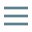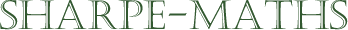# Glossary of terms

## O

•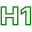### Of

•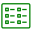Of always means the same as $\times$. In mathematics it is a synonym for multiplication. Think of it as being short for lots of.

•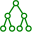$2 \ lots \ of \ 3 = 6$

$\frac{1}{4} \ of \ 20 = 5$.

•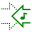•### Operations

••••### Order

•Another synonym (not very common) for index or power.
•The order of a cubic polynomial is 3, because the highest power in a cubic expression is 3.
•